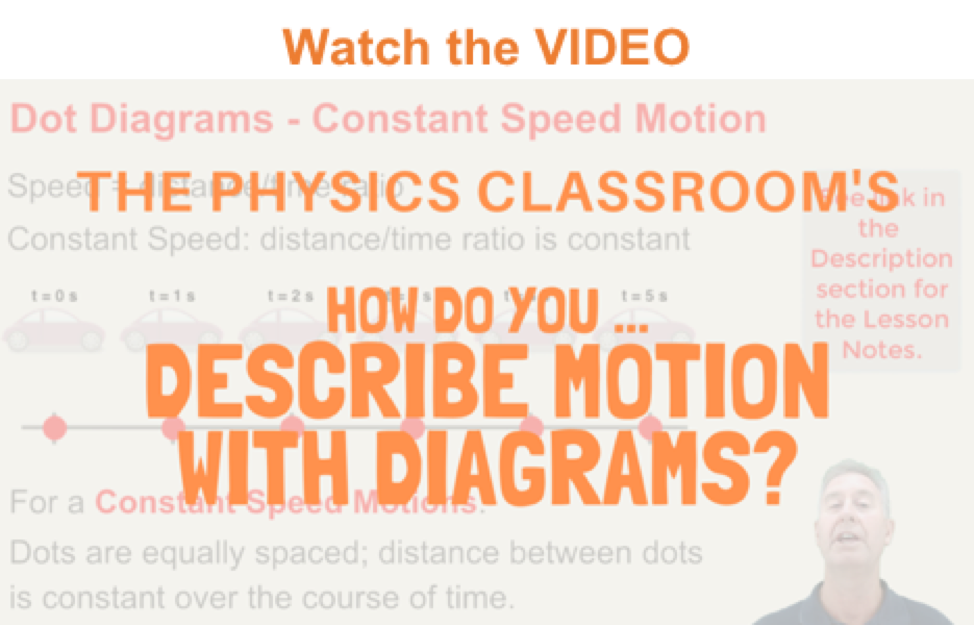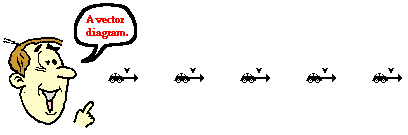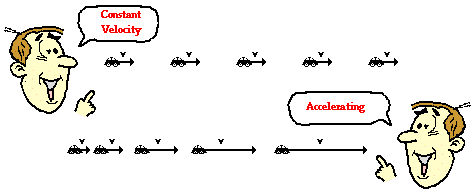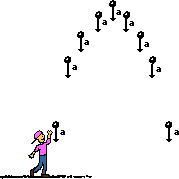1-D Kinematics - Lesson 2 - Describing Motion with Diagrams

# Vector DiagramsVector diagrams are diagrams that depict the direction and relative magnitude of a vector quantity by a vector arrow. Vector diagrams can be used to describe the velocity of a moving object during its motion. For example, a vector diagram could be used to represent the motion of a car moving down the road.In a vector diagram, the magnitude of a vector quantity is represented by the size of the vector arrow. If the size of the arrow in each consecutive frame of the vector diagram is the same, then the magnitude of that vector is constant. The diagrams below depict the velocity of a car during its motion. In the top diagram, the size of the velocity vector is constant, so the diagram is depicting a motion of constant velocity. In the bottom diagram, the size of the velocity vector is increasing, so the diagram is depicting a motion with increasing velocity - i.e., an acceleration.Vector diagrams can be used to represent any vector quantity. In future studies, vector diagrams will be used to represent a variety of physical quantities such as acceleration, force, and momentum. Be familiar with the concept of using a vector arrow to represent the direction and relative size of a quantity. It will become a very important representation of an object's motion as we proceed further in our studies of the physics of motion.